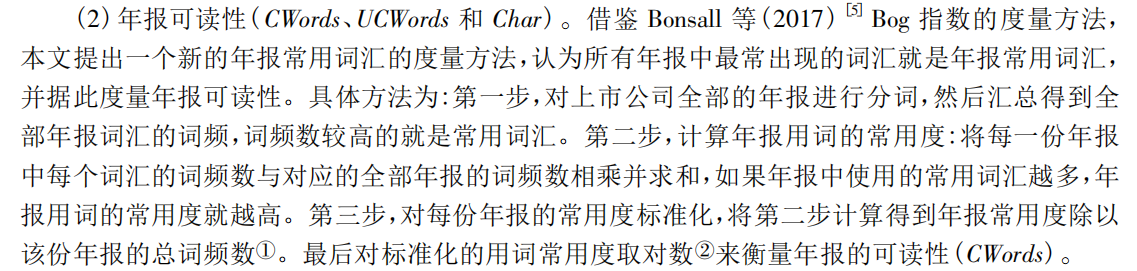###### 九个核桃

2020-12-27 20:19 阅读 15

# 如何用python实现如下文本分析？

25• 点赞
• 写回答
• 关注问题
• 收藏
• 复制链接分享

#### 5条回答默认 最新

• 已采纳
``````import math
import jieba
###这里是年报中的内容，如果需要读pdf 文件可加一段就可以了，这里给你个样例试跑结果
wjj=['我在北京','我在南京','我在巴黎年报北京我在南京']

def getdic(str1):
a=jieba.lcut(str1)
dic1={}
for i in a:
if i in dic1:
dic1[i]+=1
else:
dic1[i]=1
return dic1

res0=[]
for i in wjj:
res0.append(getdic(i))

dics={}
for j in res0:
for k in j:
if k not in dics:
dics[k]=j[k]
else:
dics[k]+=j[k]

slist=[]
for m in res0:
sm=0
for n in m:
sm+=m[n]*dics[n]
slist.append((sm,sum(m.values())))

##这里对数用的e ，不知道文章中需要什么对数
res=[math.log(j1/j2) for j1,j2 in slist]
print(res)``````
点赞 1 评论 复制链接分享
• 你要读取什么？输出什么？

点赞 评论 复制链接分享
• 就是分析年报的可读性，指标是CWords  按照上面1.2.3步，最后求出来每一份年报的值并输出

点赞 评论 复制链接分享
• 大佬，能不能最后输出每份年报和它对应的最终值？最后形成一个EXCEL或者csv，第一列是年报名称，第二列是对应的值

点赞 评论 复制链接分享
• 当然可以，你的pdf 文本是否需要我在代码里处理。如果需要你的pdf 是否在一个文件夹里，我按文件夹读取就可以。还有看下文中的注释，对数是多少为底的对数。

点赞 评论 复制链接分享